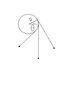# Lagrangian function of pendulum

• Grand

## Homework Statement

Find the Lagrangian and the Lagrangian equations for this pendulum (see the picture). Radius of circle is a, mass of the bob is m and l is the length of the pendulum when it hangs straight down.

## The Attempt at a Solution

I obtain:
$$L=\frac{1}{2}m(a^2\dot{\theta}^2+(l+a\theta)^2\dot{\theta}^2)-mg(a\sin\theta-(l+a\theta)\cos\theta)$$
while the answer in the book misses the term $$a^2\dot{\theta}^2$$ - where have I gone wrong?

#### Attachments

•pendulum.jpg
4.1 KB · Views: 413
How did you get that extra term? The x,y coordinates of the bob with respect to the centre of the circle are x=a cos(θ)+(l+a)sin(θ), y=sin(θ)-(l+a)cos(θ). Take the time derivatives to get the x, y components of velocity.

ehild